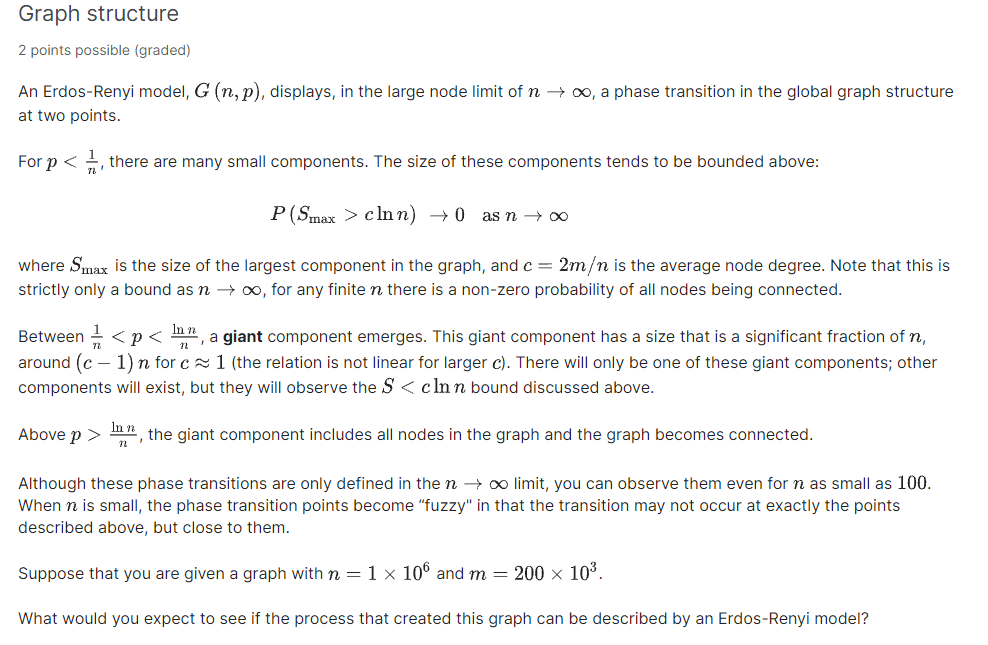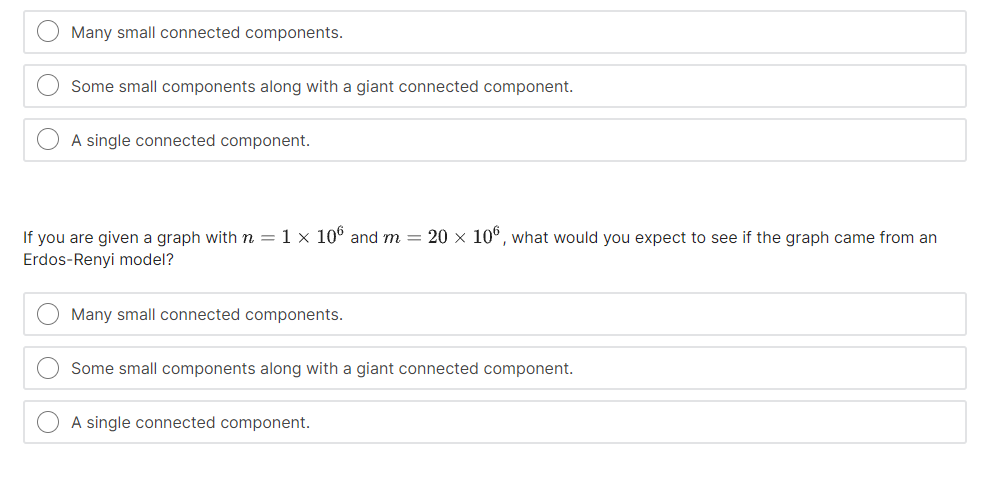# Question Graph structure 2 points possible (graded) An Erdos-Renyi model, G (n,p), displays, in the large node limit of n +o, a phase transition in the global graph structure at two points. For p < , there are many small components. The size of these components tends to be bounded above: P(Smax > clnn) → as n + where Smax is the size of the largest component in the graph, and c = 2m/n is the average node degree. Note that this is strictly only a bound as n +, for any finite n there is a non-zero probability of all nodes being connected. 12 Between 1 <p < Inn, a giant component emerges. This giant component has a size that is a significant fraction of n, around (C – 1)n for c = 1 (the relation is not linear for larger c). There will only be one of these giant components; other components will exist, but they will observe the S<cln n bound discussed above. Inn Above p > the giant component includes all nodes in the graph and the graph becomes connected. 12 Although these phase transitions are only defined in the n → limit, you can observe them even for n as small as 100. When n is small, the phase transition points become "fuzzy" in that the transition may not occur at exactly the points described above, but close to them. Suppose that you are given a graph with n=1 x 106 and m = 200 x 103. What would you expect to see if the process that created this graph can be described by an Erdos-Renyi model? Many small connected components. Some small components along with a giant connected component. A single connected component. If you are given a graph with n=1 x 106 and m = 20 x 106, what would you expect to see if the graph came from an Erdos-Renyi model? Many small connected components. Some small components along with a giant connected component. A single connected component.QQD5JP The Asker · Computer ScienceTranscribed Image Text: Graph structure 2 points possible (graded) An Erdos-Renyi model, G (n,p), displays, in the large node limit of n +o, a phase transition in the global graph structure at two points. For p < , there are many small components. The size of these components tends to be bounded above: P(Smax > clnn) → as n + where Smax is the size of the largest component in the graph, and c = 2m/n is the average node degree. Note that this is strictly only a bound as n +, for any finite n there is a non-zero probability of all nodes being connected. 12 Between 1

the giant component includes all nodes in the graph and the graph becomes connected. 12 Although these phase transitions are only defined in the n → limit, you can observe them even for n as small as 100. When n is small, the phase transition points become "fuzzy" in that the transition may not occur at exactly the points described above, but close to them. Suppose that you are given a graph with n=1 x 106 and m = 200 x 103. What would you expect to see if the process that created this graph can be described by an Erdos-Renyi model? Many small connected components. Some small components along with a giant connected component. A single connected component. If you are given a graph with n=1 x 106 and m = 20 x 106, what would you expect to see if the graph came from an Erdos-Renyi model? Many small connected components. Some small components along with a giant connected component. A single connected component.

More
Transcribed Image Text: Graph structure 2 points possible (graded) An Erdos-Renyi model, G (n,p), displays, in the large node limit of n +o, a phase transition in the global graph structure at two points. For p < , there are many small components. The size of these components tends to be bounded above: P(Smax > clnn) → as n + where Smax is the size of the largest component in the graph, and c = 2m/n is the average node degree. Note that this is strictly only a bound as n +, for any finite n there is a non-zero probability of all nodes being connected. 12 Between 1

the giant component includes all nodes in the graph and the graph becomes connected. 12 Although these phase transitions are only defined in the n → limit, you can observe them even for n as small as 100. When n is small, the phase transition points become "fuzzy" in that the transition may not occur at exactly the points described above, but close to them. Suppose that you are given a graph with n=1 x 106 and m = 200 x 103. What would you expect to see if the process that created this graph can be described by an Erdos-Renyi model? Many small connected components. Some small components along with a giant connected component. A single connected component. If you are given a graph with n=1 x 106 and m = 20 x 106, what would you expect to see if the graph came from an Erdos-Renyi model? Many small connected components. Some small components along with a giant connected component. A single connected component.